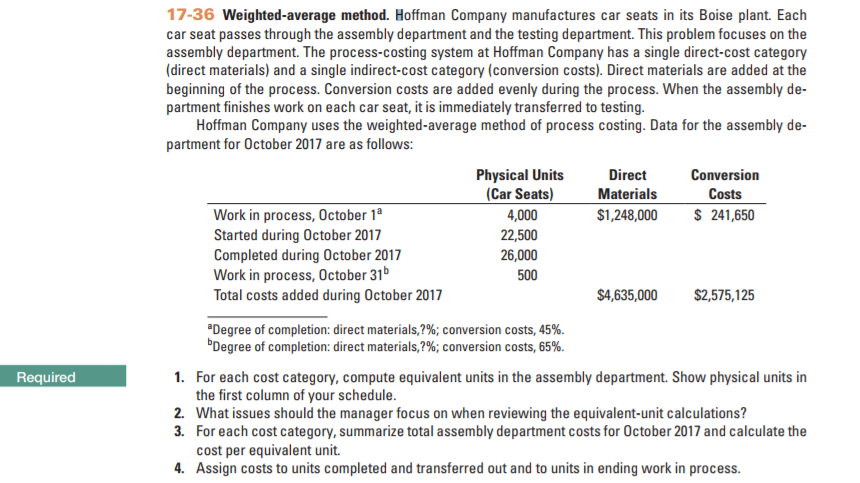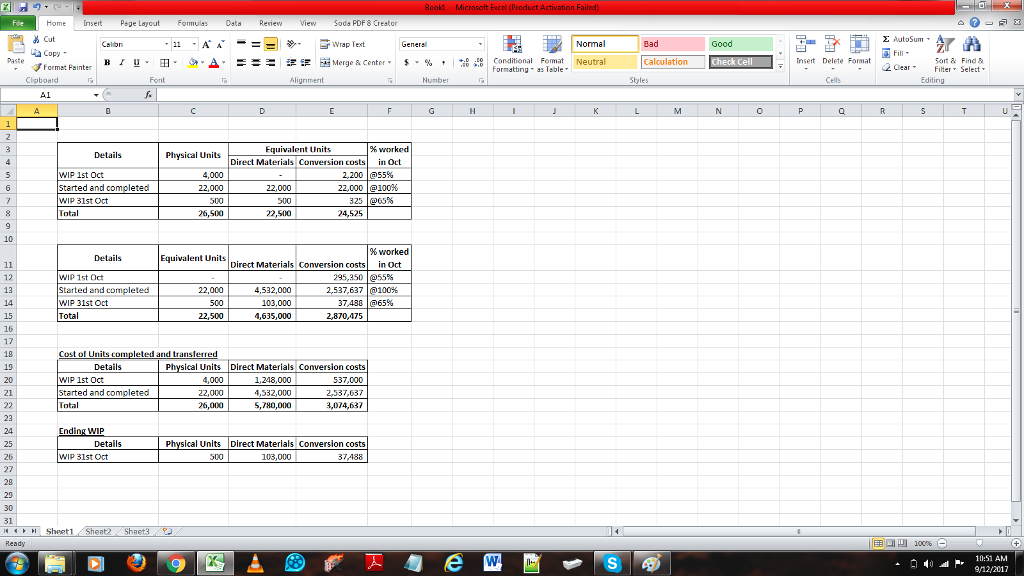# Question & Answer: Hello, I was looking for the answers of the exercice. Thank you very much for the help !…..Hello, I was looking for the answers of the exercice. Thank you very much for the help !

Don't use plagiarized sources. Get Your Custom Essay on
Question & Answer: Hello, I was looking for the answers of the exercice. Thank you very much for the help !…..
GET AN ESSAY WRITTEN FOR YOU FROM AS LOW AS \$13/PAGE

Hoffman Company manufactures car seats in its Boise plant. Each car seat passes through the assembly department and the testing department. This problem focuses on the assembly department. The process-costing system at Hoffman Company has a single direct-cost category (direct materials) and a single indirect-cost category (conversion costs). Direct materials are added at the beginning of the process. Conversion costs are added evenly during the process. When the assembly department finishes work on each car seat, it is immediately transferred to testing. Hoffman Company uses the weighted-average method of process costing. Data for the assembly department for October 2017 are as follows: For each cost category, compute equivalent units in the assembly department. Show physical units in the first column of your schedule. What issues should the manager focus on when reviewing the equivalent-unit calculations? For each cost category, summarize total assembly department costs for October 2017 and calculate the cost per equivalent unit. Assign costs to units completed and transferred out and to units in ending work in process.

1) Equivalent units is computed based on % completion of costs depending on the different stages of the process.

As direct materials are added in the beginning of the process, it will be taken as 100% completion at any given stage

Separate completion % for conversion costs are given, i.e. 45% completion for the opening WIP, which means, 45% of the conversion for the opening WIP units were completed in the previous period and remaining completion will be done during October. The same have to be deducted from the completed units in October

Based on the above logic, the calculation of equivalent units is the picture attached:

2) When reviewing the equivalent units, the maanger should focus on quality control, costs of completion, damages occured preventing the % of completed units to be transferred, costs of reworking, etc, to ensure optimum use of resources

3) Total costs for assembly department for October is calculated based on the equivalent units as shown in the image. No costs are considered for opening WIP direct materials as they have been considered in the previous period itself. All costs for closing WIP direct materials have been considered during this month as the materials are already added to the process.

for conversion costs, as 45% was converted in previous month, we take 55% being the remaining conversion based on extrapolated amounts as per opening balance. 241650 for 45% equates to 295350 for 55%

For units started and completed during the month, which is 22000, we take 100% based on the total costs for Oct, i.e. 2575125/22325 x 22000 = 2537637.

22325 is the equivalent unit calculated earlier.

For closing WIP, as 65% have already been worked upon, we consider 65% of the above per unit costs, i.e. 2575125/22325 x 325 = 37488. 325 being the equivalent units for 65% completion

4) Costs for units completed and transferred out as well as ending work in progress is calculated as shown in the image

Completed and transferred –

Direct Materials:

direct materials opening WIP + costs incurred during Oct(as per logic above, i.e. direct materials @100%)

=1248000+4532000 = 5780000

Conversion costs:

Opg WIP + costs for remaining conversion for opening WIP units + conversion costs for fully completed units in Oct + costs for % completed as part of closing WIP

=241650+295350+2537637(as per 3) = 3074637

Ending WIP:

Direct materials = 103000, as arrived in 3) above

Conversion costs = 37488, as arrived in 3) above